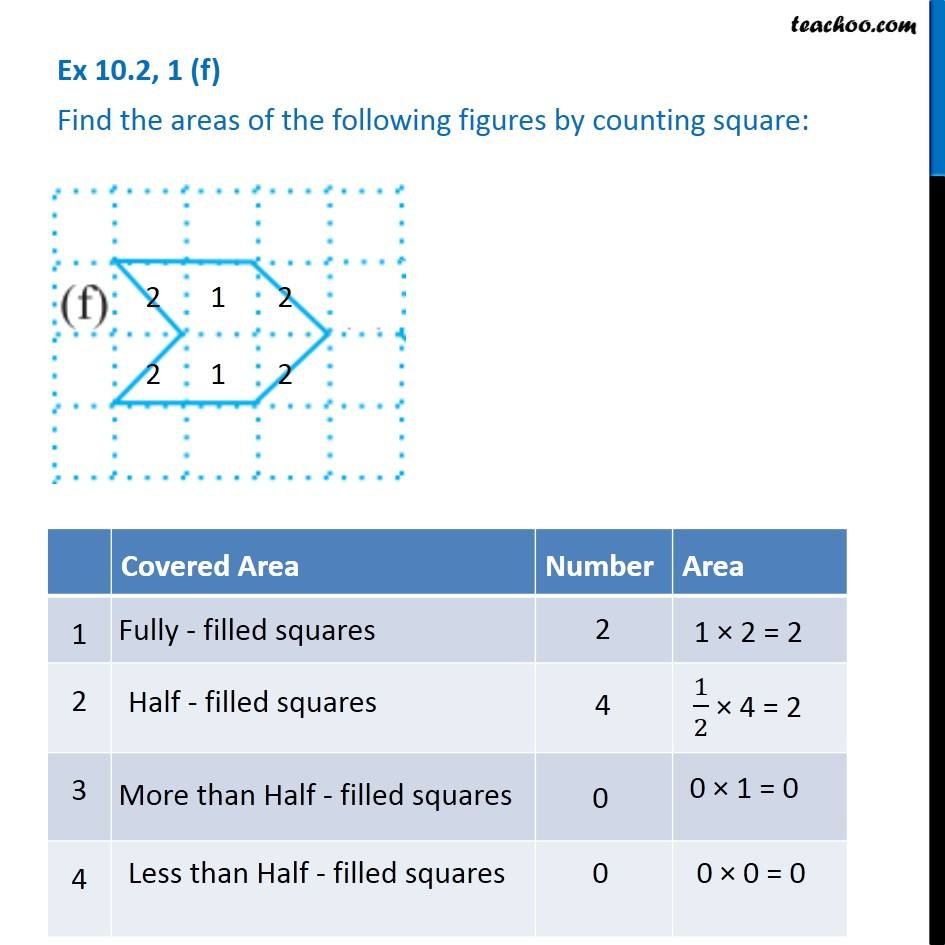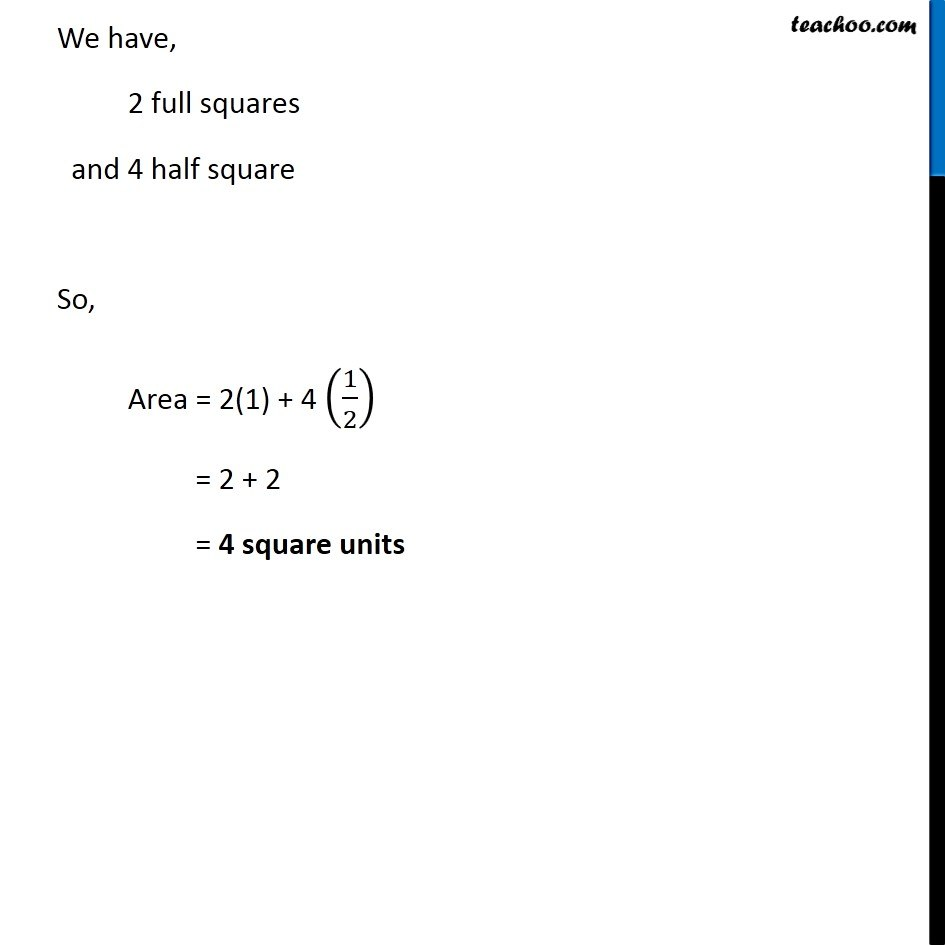Ex 10.2

Chapter 10 Class 6 Mensuration
Serial order wiseGet live Maths 1-on-1 Classs - Class 6 to 12

### Transcript

Ex 10.2, 1 (f) Find the areas of the following figures by counting square: Covered Area Number Area 1 Fully filled squares 2 1 × 2 = 2 2 Half filled squares 4 1/2 × 4 = 2 3 More than Half filled squares 0 0 × 1 = 0 4 Less than half filled squares 0 0 × 0 = 0 We have, 2 full squares and 4 half square So, Area = 2(1) + 4 (1/2) = 2 + 2 = 4 square units